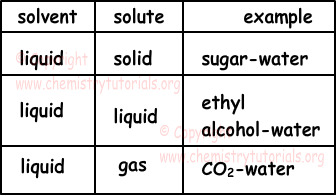## Solutions

Solutions

As we mention in previous topics, solutions are homogeneous mixtures of two or more than two matters. Solutions can be in solid, liquid and gas phases. Example of gas phase solutions; air, liquid phase solutions; salt+water mixtures, solid phase solutions; Zn-Cu alloy. Solutions has two components, solute and solvent. Solvent is the medium in which matter is dissolved and solute is the matter that dissolves in solvent. For example, in water sugar solution, water is the solvent that dissolves sugar and sugar is the solute that is dissolved in water. If there are more than two matters in solution, then matter having larger magnitude becomes solvent of the solution and others are solute. How ever, there are some exceptions, in solid-liquid solutions amount of solid can be larger than amount of liquid but we assume liquid as solvent. Table given below shows example of liquid, gas and solid solutions in which liquid is solvent.Dilute Solution: Solutions having small amount of solute in solvent are called dilute solutions.

Concentrated Solution: Solutions in which large amount of solute dissolves in solvent.

These concepts are used in comparing two solutions.Read following statements, they will help you in problem solving.

• Mass of solution is equal to sum of masses of solvent and solute.
• In solid-liquid solutions, volume of solution is larger than the volume of solvent.
• In liquid-liquid solutions, volume of solution can be larger than volumes of sum of solute and solvent. Chemical properties of matters forming solution should be known to talk about volume of solution.

Solvation: It is the process of dissolution of solute in solvent.

Types of Solvation:

1) Ionic Solvation: If solvent decomposes into its ions, we call these solutions ionic solutions. Acids, bases and salts produce ionic solutions. Since these solutions include ions, they conduct electricity. Examples of ionic solutions are given below;

NaNO3(s) → Na+(aq) + NO3-(aq)

(NH4)2SO4(s) → 2NH4+(aq) + SO4-2(aq)

Mg(NO3)2(s) → Mg+2(aq) + 2NO3-(aq)

NaCl(s) → Na+(aq) + Cl-(aq)

2) Molecular Solvation: If a matter decomposes into its molecules, we call these solutions molecular solutions. Dissolution of sugar in water is example of molecular solvation. Since there is no ion in structure of these solutions, they do not conduct electricity. Examples of molecular solvation are given below;

C6H12O6(s) → C2H12O6(aq)

glucose

O2(g) → O2(aq)

C2H5OH(l) → C2H5OH(aq)

alcohol

We can examine solutions under two title according to their saturation ratio; such as saturated solutions and unsaturated solutions and supersaturated solutions.

1) Saturated Solutions: If solution dissolves maximum amount of solute at specific temperature, then we call them as saturated solutions. In this type of solutions there can be solid matters (undissolved) at the bottom of tank.2) Unsaturated Solutions: If solutions can solve more solute at specific temperature, then we cal them unsaturated solutions. If you vaporize some of the solvent or add some solute you can make them saturated solutions.

3) Supersaturated Solutions: If solutions contain more solute than its capacity, we call these solutions supersaturated solutions. We prepare them by heating solution and adding solute, after that we cool slowly supersaturated solution. You can observe crystallization of solute in supersaturated solutions.

Solutions Exams and  Problem Solutions

Related